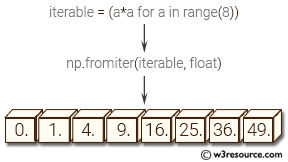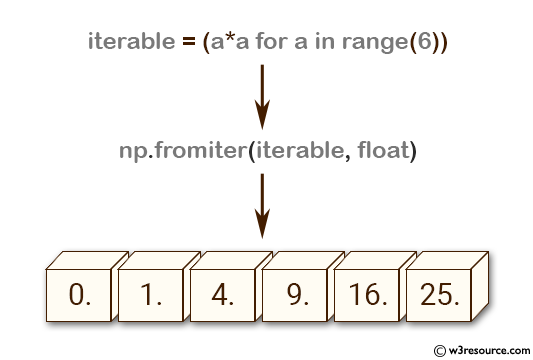# NumPy: numpy.fromiter() function

## numpy.fromiter() function

The numpy.fromiter() function is used to create a new 1-dimensional array from an iterable object.

The fromiter() function is useful when we want to create a new numpy array from an iterable object without having to convert the iterable to a list or tuple first. It is also useful when we have a large dataset and we do not want to load it all into memory at once. Instead, we can read it in chunks using a generator and create a new numpy array from each chunk using fromiter().

Syntax:

`numpy.fromiter(iterable, dtype, count=-1)`Parameters:

Name Description Required /
Optional
iterable An iterable object providing data for the array. Required
dtype The data-type of the returned array. Required
count The number of items to read from iterable. The default is -1, which means all data is read. Optional

Return value:

out : ndarray
The output array.

Example: Creating NumPy array from iterable using fromiter()

``````>>> import numpy as np
>>> iterable = (a*a for a in range(6))
>>> np.fromiter(iterable, float)
array([  0.,   1.,   4.,   9.,  16.,  25.])
``````

In the above code, an iterable object is created using a generator expression that yields the squares of integers from 0 to 5.
The fromiter() function is then called with the iterable object and the data type of the resulting array as arguments. It returns a new one-dimensional NumPy array containing the values yielded by the iterable object, converted to the specified data type.
In this case, the resulting array is of type float and contains the first six perfect squares: 0.0, 1.0, 4.0, 9.0, 16.0, and 25.0.

Pictorial Presentation:Python - NumPy Code Editor:

Previous: fromfunction()
Next: fromstring()

﻿